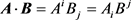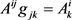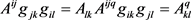# 张量概念的一种简单引入A Simple Way to Introduce the Concept of Tensor

DOI: 10.12677/MP.2019.96032, PDF, HTML, XML, 下载: 493  浏览: 1,385

Abstract: Starting from the simple Schmidt orthogonalization in mathematics, the coordinate transformation in physics is taken as the key point, and the concept of tensor is introduced simply without losing mathematical rigor and physical significance, and the operation of tensor is introduced. The purpose is to reduce the difficulty of tensor teaching in senior and graduate students of physics department.

1. 前言

2. 施密特正交化与张量概念的引入

${e}^{1}=\frac{{e}_{2}×{e}_{3}}{{e}_{1}\cdot \left({e}_{2}×{e}_{3}\right)}$

${e}^{2}=\frac{{e}_{3}×{e}_{1}}{{e}_{1}\cdot \left({e}_{2}×{e}_{3}\right)}$

${e}^{3}=\frac{{e}_{1}×{e}_{2}}{{e}_{1}\cdot \left({e}_{2}×{e}_{3}\right)}$

$k=a{e}_{1}+b{e}_{2}+c{e}_{3}$ (1)

$k\cdot {e}^{1}=a{e}^{1}\cdot {e}_{1}=a$

$k\cdot {e}^{2}=b{e}^{2}\cdot {e}_{2}=b$

$k\cdot {e}^{3}=c{e}^{3}\cdot {e}_{3}=c$

$k=\left(k\cdot {e}^{1}\right){e}_{1}+\left(k\cdot {e}^{2}\right){e}_{2}+\left(k\cdot {e}^{3}\right){e}_{3}$

$⇒$ $k={k}^{1}{e}_{1}+{k}^{2}{e}_{2}+{k}^{3}{e}_{3}$ (2)

$k={k}^{i}{e}_{i}$ (3)

${{e}^{\prime }}_{i}=\left({{e}^{\prime }}_{i}\cdot {e}^{j}\right){e}_{j}={\beta }_{i}^{j}{e}_{j}$ (4)

${{x}^{\prime }}_{i}=\left({x}_{j}{e}^{j}\right)\cdot {{e}^{\prime }}_{i}=\left({{e}^{\prime }}_{i}\cdot {e}^{j}\right){x}_{j}={\beta }_{i}^{j}{x}_{j}$ (5)

(其中 ${\beta }_{i}^{j}={{e}^{\prime }}_{i}\cdot {e}^{j}$ )

${{e}^{\prime }}^{i}=\left({{e}^{\prime }}_{i}\cdot {e}_{j}\right){e}^{j}={\left({\beta }^{-1}\right)}_{j}^{i}{e}^{j}$ (6)

${{x}^{\prime }}^{i}=\left({x}^{j}{e}_{j}\right)\cdot {{e}^{\prime }}^{i}=\left({{e}^{\prime }}^{i}\cdot {e}_{j}\right){x}^{j}={\left({\beta }^{-1}\right)}_{j}^{i}{x}^{j}$ (7)

$r=r\left({y}^{1},{y}^{2},{y}^{3}\right)={y}^{i}{\epsilon }_{i}$

$r=r\left({y}^{1},{y}^{2},{y}^{3}\right)={x}^{i}{e}_{i}$

$\text{d}r=\frac{\partial r}{\partial {y}^{i}}\text{d}{y}^{i}=\left(\frac{\partial {x}^{j}}{\partial {y}^{i}}{e}_{j}\right)\text{d}{y}^{i}$

${\epsilon }_{i}=\frac{\partial r}{\partial {y}^{i}}=\frac{\partial {x}^{j}}{\partial {y}^{i}}{e}_{j}$ (8)

${\epsilon }^{i}=\nabla {y}^{i}=\frac{\partial {y}^{i}}{\partial {x}^{j}}{e}_{j}$ (9)

${\epsilon }_{i}\cdot {\epsilon }^{k}=\frac{\partial {x}^{j}}{\partial {y}^{i}}{e}_{j}\cdot \frac{\partial {y}^{k}}{\partial {x}^{l}}{e}_{l}=\frac{\partial {x}^{j}}{\partial {y}^{i}}\frac{\partial {y}^{k}}{\partial {x}^{l}}{e}_{j}\cdot {e}_{l}=\frac{\partial {x}^{j}}{\partial {y}^{i}}\frac{\partial {y}^{k}}{\partial {x}^{l}}{\delta }_{jl}=\frac{\partial {x}^{j}}{\partial {y}^{i}}\frac{\partial {y}^{k}}{\partial {x}^{j}}={\delta }_{i}^{k}$

${\epsilon }_{i}=\frac{\partial r}{\partial {y}^{i}}$${\epsilon }^{i}=\frac{\partial r}{\partial {y}_{i}}$ (10)

1) 零阶张量：零阶张量有 ${n}^{0}=1$ 个元素且其数值与坐标变换无关，即： ${\varphi }^{\prime }=\varphi$。例如基础物理中的时间t，其不随坐标变换而发生变化。

2) 一阶张量：一阶张量有 ${n}^{1}=n$ 个元素，在坐标变换下分为一阶协变张量与一阶逆变张量

3) 二阶张量：二阶张量有 ${n}^{2}$ 个元素，在坐标变换下分为二阶协变张量，二阶逆变张量与二阶混合张量。

4) 高阶张量：有 ${n}^{p+q}$ 个元素，在一般物理理论之中不常用，故这里从略。

${C}_{{i}_{1}{i}_{2}\cdots {i}_{p}}^{{j}_{1}{j}_{2}\cdots {j}_{p}}={A}_{{i}_{1}{i}_{2}\cdots {i}_{p}}^{{j}_{1}{j}_{2}\cdots {j}_{p}}±{B}_{{i}_{1}{i}_{2}\cdots {i}_{p}}^{{j}_{1}{j}_{2}\cdots {j}_{p}}$

$\because {\epsilon }^{i}={\epsilon }^{i}\left({\epsilon }^{j}\cdot {\epsilon }_{j}\right)=\left({\epsilon }^{i}\cdot {\epsilon }^{j}\right){\epsilon }_{j}={g}^{ij}{\epsilon }_{j}$

${\epsilon }_{i}={\epsilon }_{i}\left({\epsilon }_{j}\cdot {\epsilon }^{j}\right)=\left({\epsilon }_{i}\cdot {\epsilon }_{j}\right){\epsilon }^{j}={g}_{ij}{\epsilon }^{j}$

${A}^{i}=A\cdot {\epsilon }^{i}={g}^{ij}A\cdot {\epsilon }_{j}={g}^{ij}{A}_{j}$

${A}_{i}=A\cdot {\epsilon }_{i}={g}_{ij}A\cdot {\epsilon }^{j}={g}_{ij}{A}^{j}$

$\therefore$ 度规张量 ${g}_{ij}$ 可以用于协变与逆变分量的相互转化。这一点并不奇怪，从度规的定义上来看我们可以形象的认为度规是刻画两个空间几何关系的一个张量，所以当然可以将协变量“换算”为逆变量。

$A\cdot B={A}^{i}{\epsilon }_{i}\cdot {B}^{j}{\epsilon }_{j}={A}^{i}{B}^{j}{g}_{ij}={A}^{i}{B}_{j}$ (11)(13)3. 结论

  于允强. 广义相对论引论[M]. 北京: 北京大学出版社, 2004.  黄克智. 张量分析[M]. 北京: 清华大学出版社, 1986.  余天庆, 熊睿. 张量分析概要及演算[M]. 北京: 清华大学出版社, 2014.  刘连涛, 郑小平. 物理学中的张量分析[M]. 北京: 科学出版社, 2008.